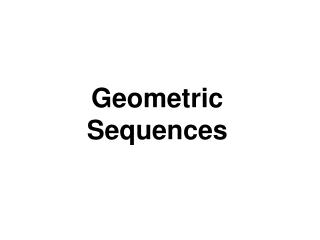DownloadDownload PresentationGeometric Sequences

# Geometric Sequences

Download Presentation## Geometric Sequences

- - - - - - - - - - - - - - - - - - - - - - - - - - - E N D - - - - - - - - - - - - - - - - - - - - - - - - - - -
##### Presentation Transcript

1. Geometric Sequences

2. Definition of a Geometric Sequence • A geometric sequence is a sequence in which each term after the first is obtained by multiplying the preceding term by a fixed nonzero constant. The amount by which we multiply each time is called the common ratio of the sequence.

3. The Common Ratio The common ratio, r, is found by dividing any term after the first term by the term that directly precedes it. In the following examples, the common ratio is found by dividing the second term by the first term, a2¸a1. Geometric sequence Common ratio 1, 5 25, 125, 625... r= 5 ¸ 1 = 5 6, -12, 24, -48, 96.... r= -12 ¸ 6 = -2

4. Text Example Write the first six terms of the geometric sequence with first term 6 and common ratio 1/3 . Solution The first term is 6. The second term is 6  1/3, or 2. The third term is 2  1/3, or2/3.The fourth term is 2/3  1/3, or2/9, and so on. The first six terms are 6, 2,2/3,2/9,2/27,2/81.

5. General Term of a Geometric Sequence • The nth term (the general term) of a geometric sequence with the first term a1 and common ratio r is • an = a1 r n-1

6. Text Example Find the eighth term of the geometric sequence whose first term is -4and whose common ratio is -2. Solution To find the eighth term, a8, we replace n in the formula with 8, a1 with -4,and r with -2. an=a1rn- 1 a8 = -4(-2)8 - 1=-4(-2)7=-4(-128) =512 The eighth term is 512. We can check this result by writing the first eight terms of the sequence: -4, 8, -16, 32, -64, 128, -256, 512.

7. The Sum of the First n Terms of a Geometric Sequence The sum, Sn, of the first n terms of a geometric sequence is given by in which a1 is the first term and r is the common ratio.

8. Example • Find the sum of the first 12 terms of the geometric sequence: 4, -12, 36, -108, ... Solution:

9. If P is the deposit made at the end of each compounding period for an annuity at r percent annual interest compounded n times per year, the value, A, of the annuity after t years: Value of an Annuity: Interest Compounded n Times per Year

10. Example • To save for retirement, you decide to deposit \$2000 into an IRA at the end of each year for the next 30 years. If the interest rate is 9% per year compounded annually, find the value of the IRA after 30 years. Solution: P=2000, r =.09, t = 30, n=1

11. Example cont. • To save for retirement, you decide to deposit \$2000 into an IRA at the end of each year for the next 30 years. If the interest rate is 9% per year compounded annually, find the value of the IRA after 30 years. Solution:

12. If -1<r<1, then the sum of the infinite geometric series a1+a1r+a1r2+a1r3+… in which a1 is the first term and r s the common ration is given by The Sum of an Infinite Geometric Series If |r|>1, the infinite series does not have a sum.

13. Geometric Sequences